# Donut chart in R with lessR

lessR

David W. Gerbing

## Donut chart with `lessR` package

Donut or doughnut charts are an alternative chart for pie charts, which have a hole in the middle, making them cleaner to read than pie charts. In base R it is possible to create this type of visualizations with `PieChart` function from `lessR` package.

Consider the `"Employee"` data (provided by the package) and pass the `Dept` (department) column to the function, indicating the name of the data set. We have set `main = NULL` to remove the default title. Note that you can also use `pc` function as a shorthand.

``````# install.packages(lessR)
library(lessR)

# Sample data of the package
data <- rd("Employee")

# Donut chart
PieChart(Dept, data = data,
main = NULL)

# Equivalent to:
pc(Dept, data = data,
main = NULL)``````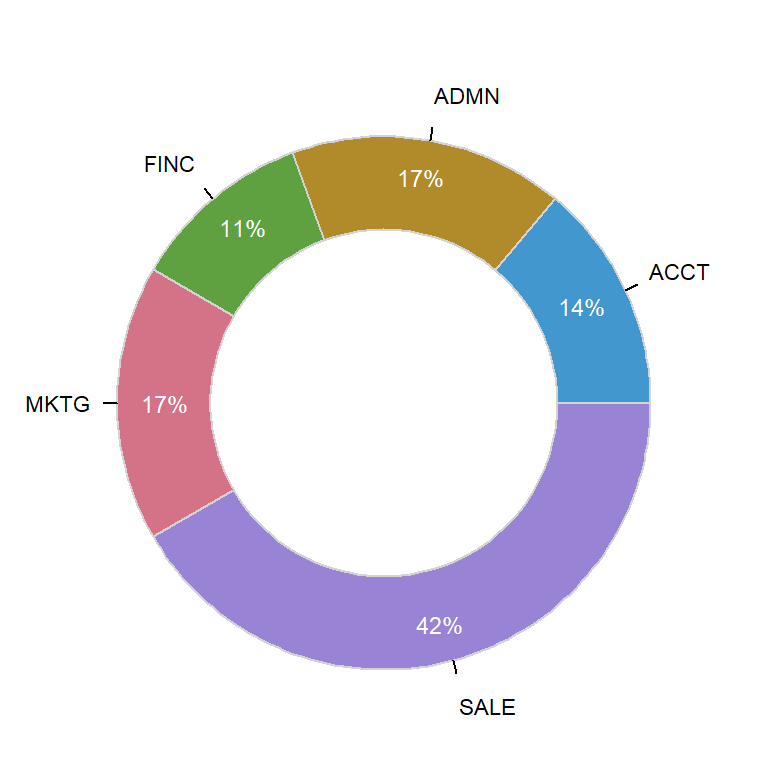The function also provides an argument named `rows`, which allows you to make a subset of your data. In this example we are selecting only females with a salary higher than 45000.

``````# install.packages(lessR)
library(lessR)

# Sample data of the package
data <- rd("Employee")

# Donut chart
PieChart(Dept, data = data,
rows = (Gender == "F" & Salary > 45000),
main = NULL)``````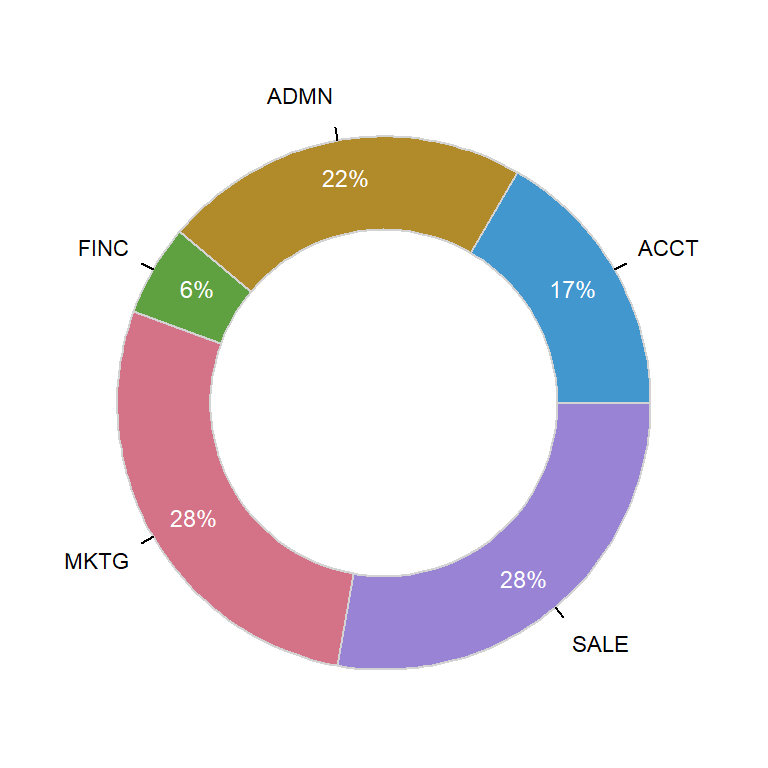### Hole sizeThe `hole` argument controls the size of the hole, from 0 (a pie chart) to 0.99. Default value is 0.65.

``````# install.packages(lessR)
library(lessR)

# Sample data of the package
data <- rd("Employee")

# Donut chart
PieChart(Dept, data = data,
hole = 0.1,
main = NULL)``````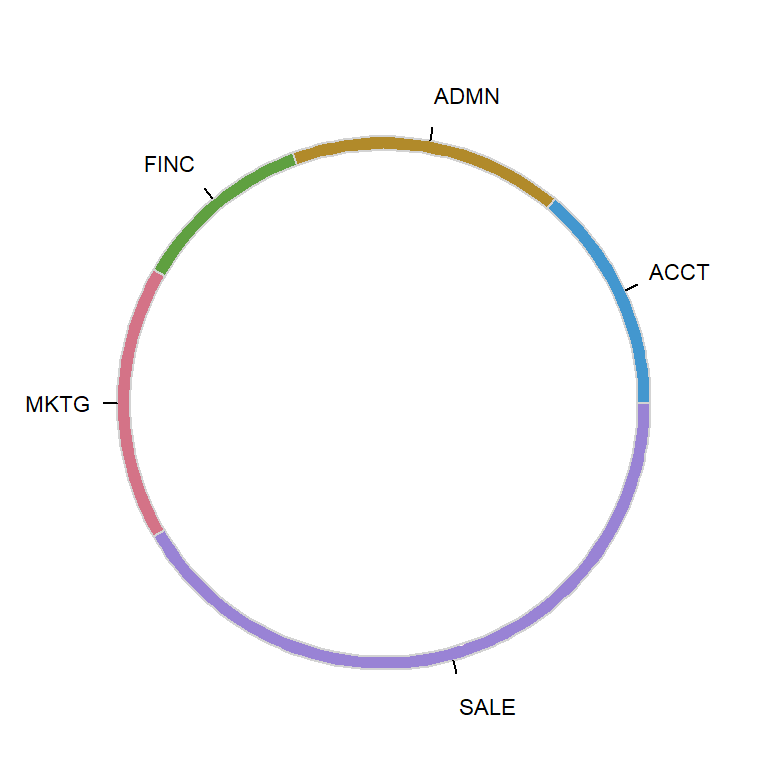If you set a value closer to 1 you can create a thick donut chart as in the example of the following block of code.

``````# install.packages(lessR)
library(lessR)

# Sample data of the package
data <- rd("Employee")

# Donut chart
PieChart(Dept, data = data,
hole = 0.95,
main = NULL)``````

### Donut colors

You can change the color of the slices with `fill`. In this example we are using the `"Zissou 1"` palette, but you can choose your own. Note that the color palette must be of the same length of the number of slices.

``````# install.packages(lessR)
library(lessR)

# Sample data of the package
data <- rd("Employee")

# Color palette
cols <- hcl.colors(length(unique(data\$Dept)), "Zissou 1")

# Donut chart
PieChart(Dept, data = data,
fill = cols,
main = NULL)``````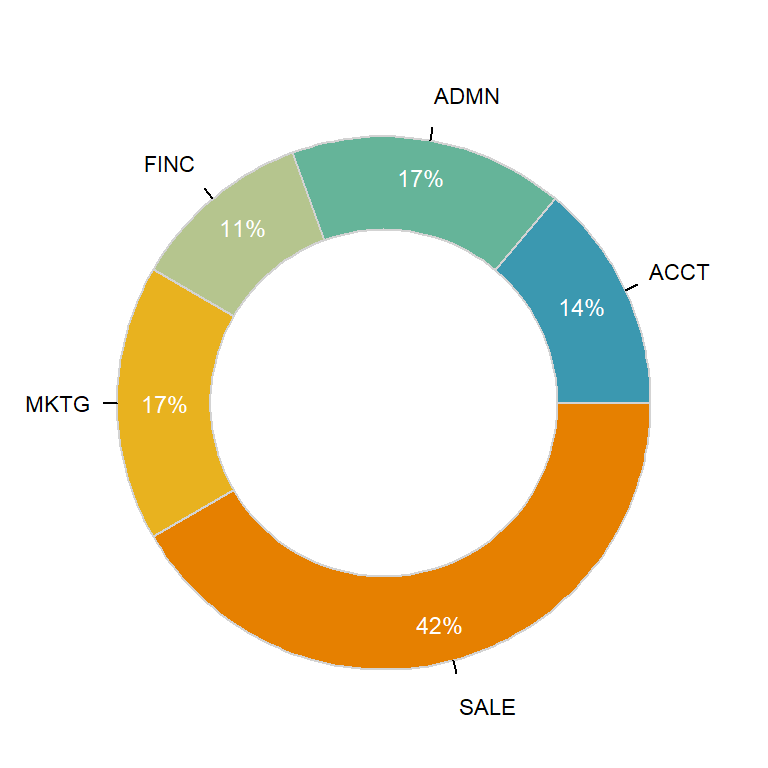The `fill` argument also admits some pre-specified color palettes, such as `"viridis"`, `"blues"` or `"heat"`, among others.

``````# install.packages(lessR)
library(lessR)

# Sample data of the package
data <- rd("Employee")

# Donut chart
PieChart(Dept, data = data,
fill = "viridis",
main = NULL)``````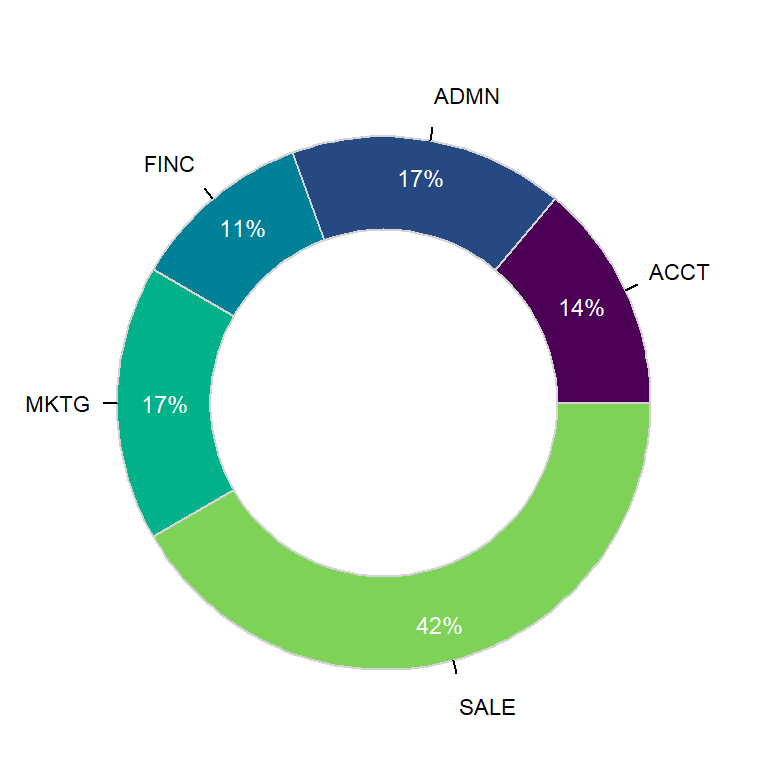The default border color (lightgray) can also be modified. Pass the desired color to `color` argument and choose a line width and type with `lwd` and `lty`.

``````# install.packages(lessR)
library(lessR)

# Sample data of the package
data <- rd("Employee")

# Donut chart
PieChart(Dept, data = data,
fill = "viridis",
color = "black",
lwd = 2,
lty = 1,
main = NULL)``````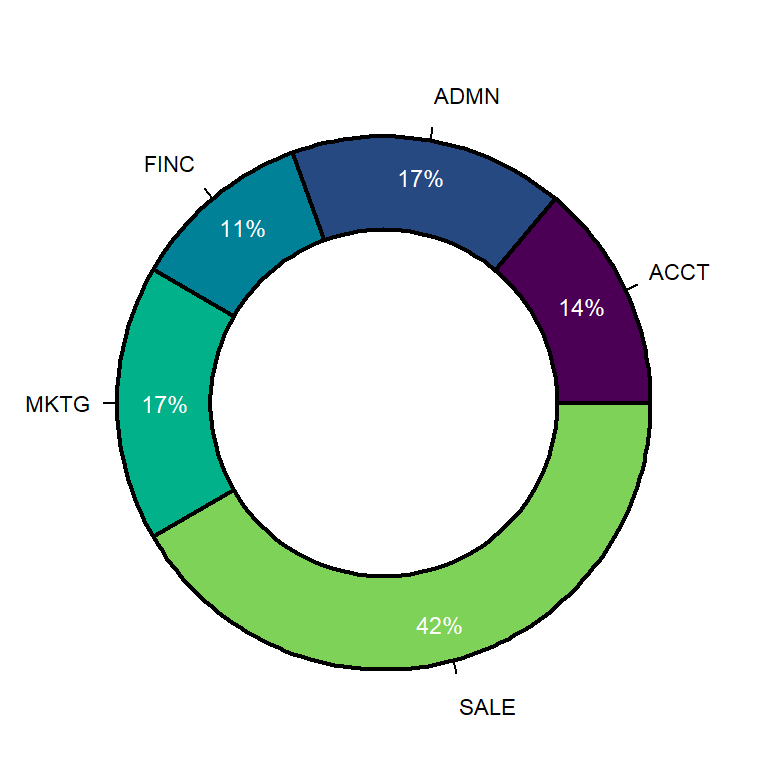You can also change the fill color of the hole, which defaults to white. This is specially useful if you change the background color of the plot.

``````# install.packages(lessR)
library(lessR)

# Sample data of the package
data <- rd("Employee")

# Donut chart
PieChart(Dept, data = data,
fill = "blues",
hole_fill = "#B7E3E0",
main = NULL)``````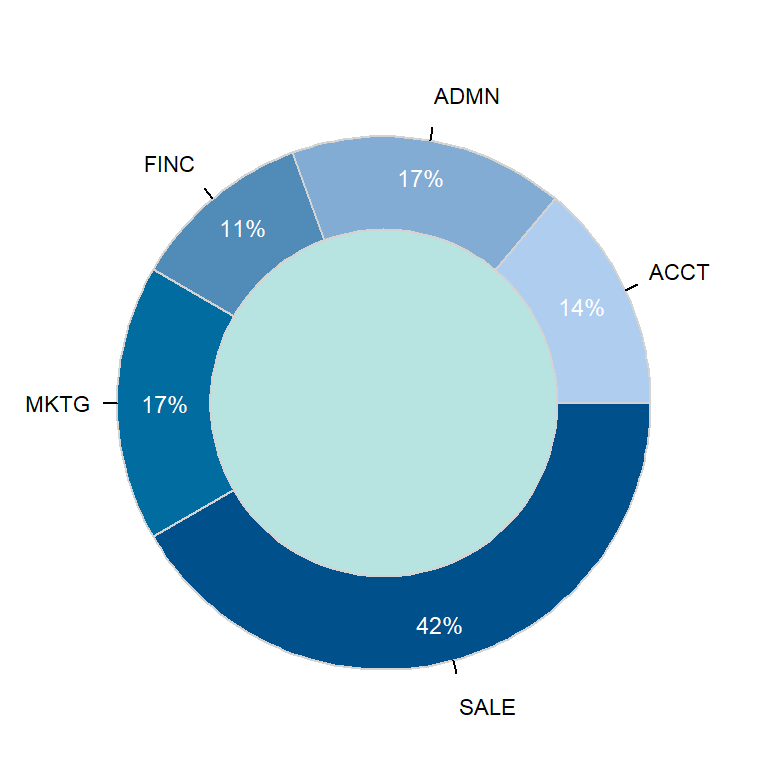### Values customization

The `labels` argument can be used to remove the values, to set them as proportions or as they are. Note that the function show percentages by default. You can also use `values_size`, `values_digits`, `values_color` and `values_position` to customize them. Possible options are the displayed below.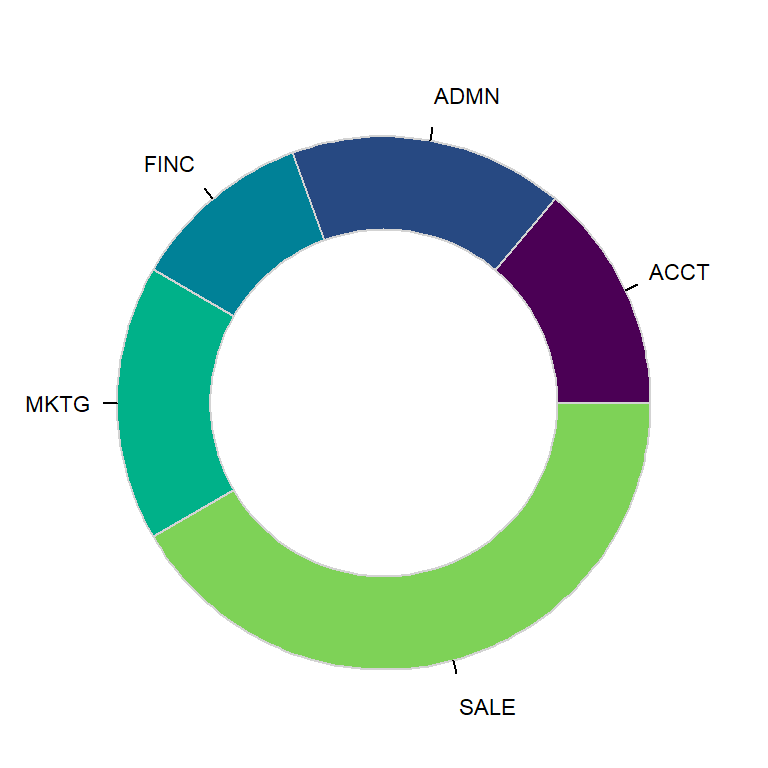Remove values

``````# install.packages(lessR)
library(lessR)

# Sample data of the package
data <- rd("Employee")

# Donut chart
PieChart(Dept, data = data,
fill = "viridis",
values = "off",
main = NULL)``````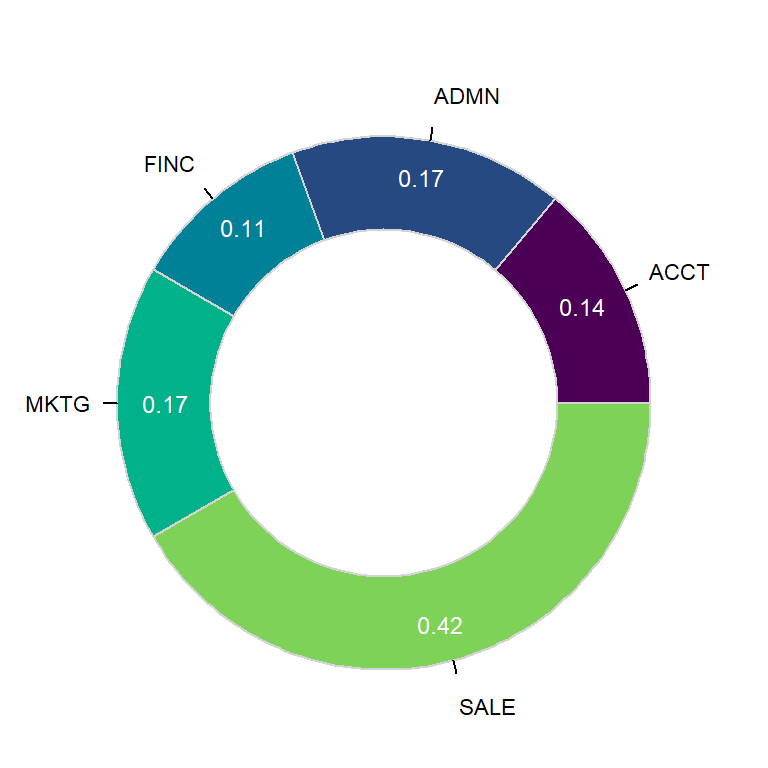Values as a proportion

``````# install.packages(lessR)
library(lessR)

# Sample data of the package
data <- rd("Employee")

# Donut chart
PieChart(Dept, data = data,
fill = "viridis",
values = "prop",
main = NULL)``````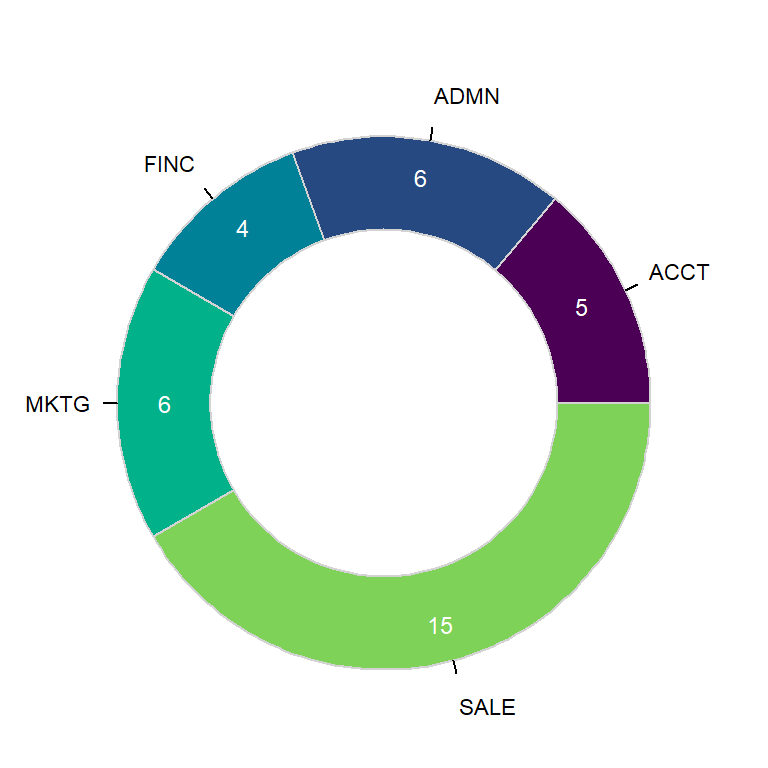Values as input data

``````# install.packages(lessR)
library(lessR)

# Sample data of the package
data <- rd("Employee")

# Donut chart
PieChart(Dept, data = data,
fill = "viridis",
values = "input",
main = NULL)``````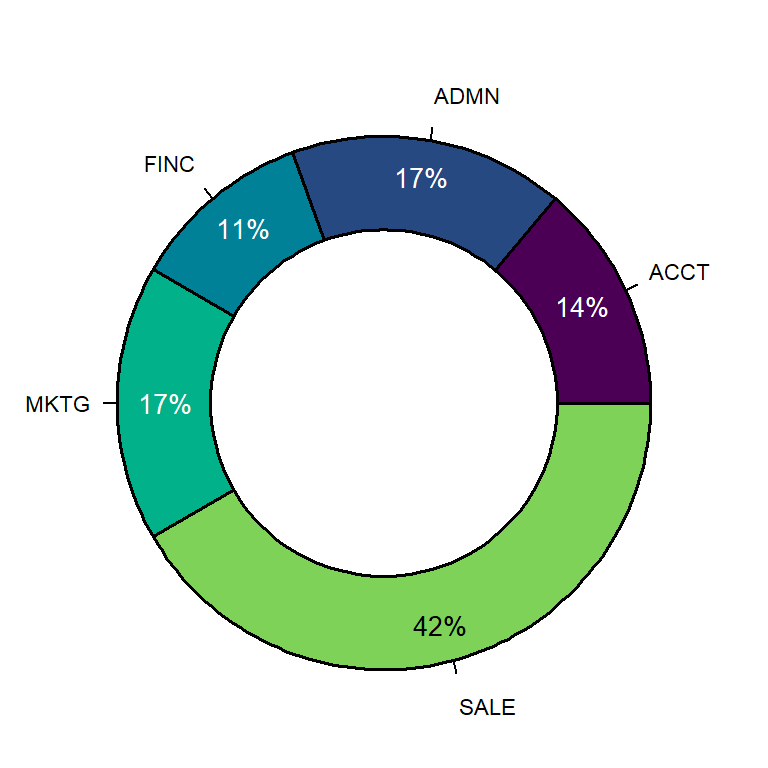Values customization

``````# install.packages(lessR)
library(lessR)

# Sample data of the package
data <- rd("Employee")

# Donut chart
PieChart(Dept, data = data,
fill = "viridis",
main = NULL,
color = "black",
lwd = 1.5,
values_color = c(rep("white", 4), 1),
values_size = 0.85)``````